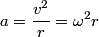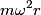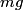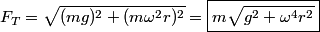## Solution to 1986 Problem 37

 The centripetal acceleration must be\begin{align*}a = \frac{v^2}{r} = \omega^2 r\end{align*}So,$m \omega^2 r$ must be the horizontal component of the tension in the cord. The mass is not accelerating in the vertical direction, so the vertical component of the tension must be$mg$. So, the tension in the cord is:\begin{align*}F_T = \sqrt{(m g)^2 + (m \omega^2 r)^2 } = \boxed{m \sqrt{g^2 + \omega^4 r^2}}\end{align*}Therefore, answer (E) is correct.